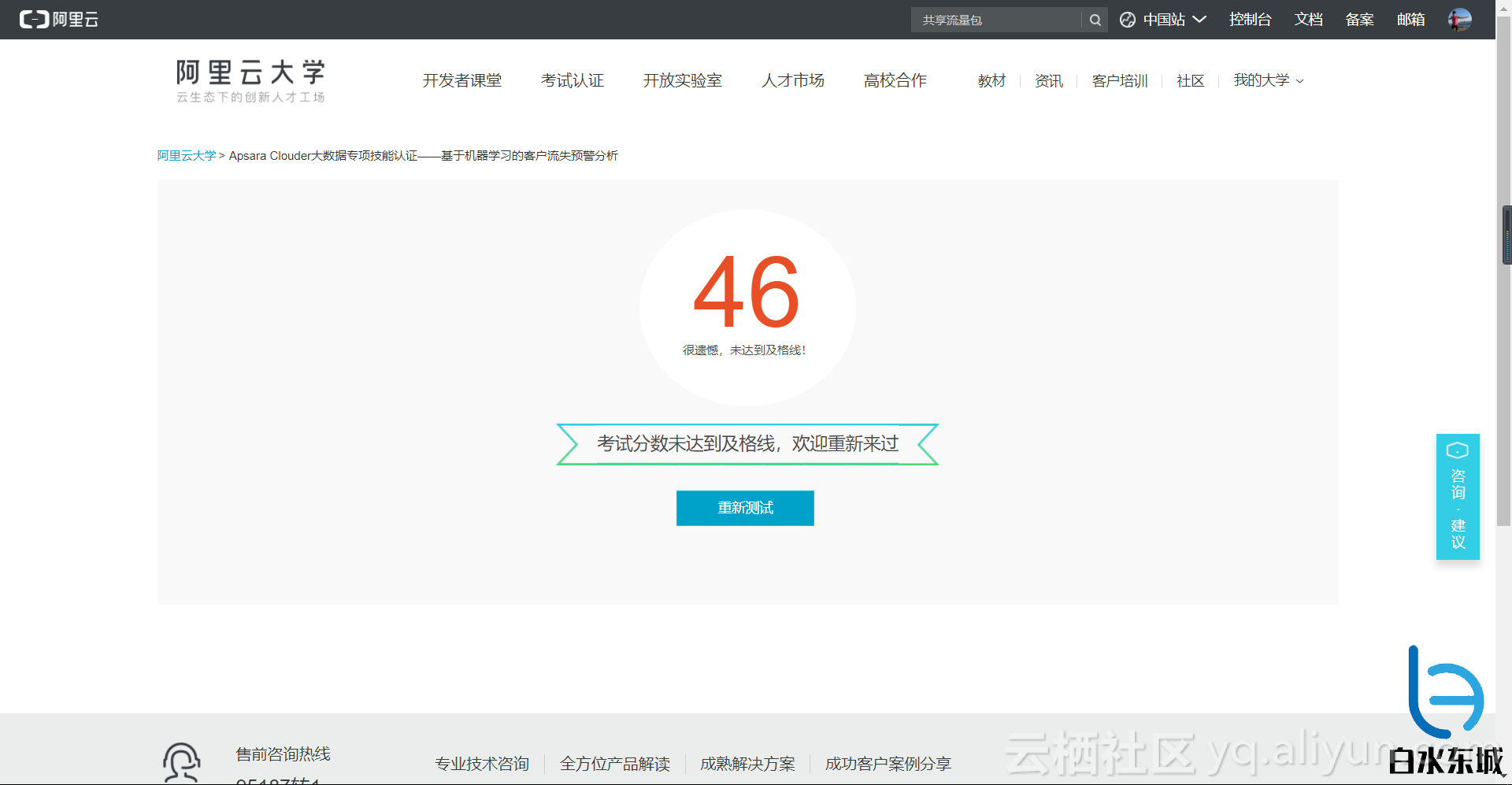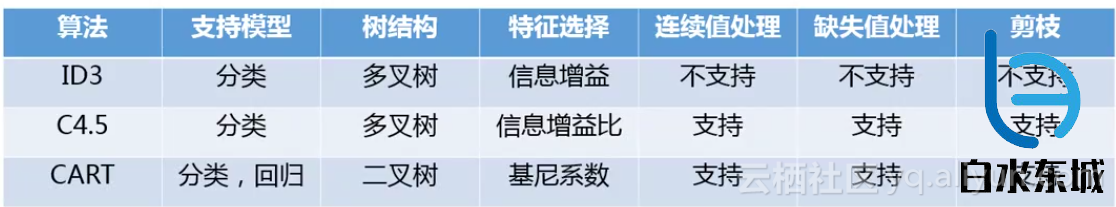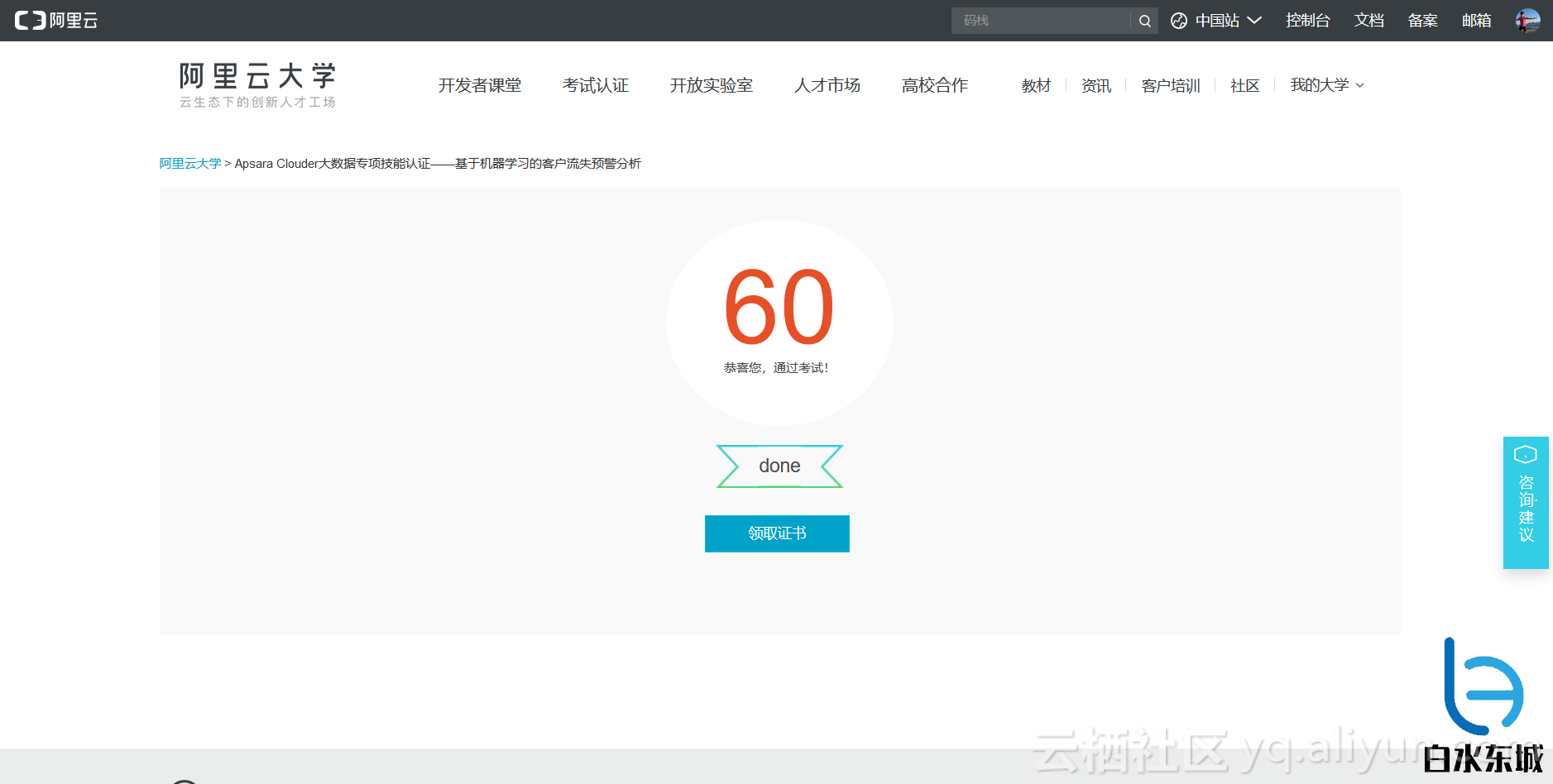#0
0
01. 云栖社区>
2. 博客>
3. 正文

## 机器学习入门|决策树（二）

kissjz 2018-02-03 23:45:02 浏览6352OK ,里面考了很多决策树的知识，想想也对，课程中唯一提到的机器学习算法就是决策树，你说考不考(ノへ￣、)

# 信息增益与ID3算法

ID3算法（Iterative Dichotomiser 3，迭代二叉树3代），是Ross Quinlan发明的一种决策树算法。

$$Ent(D)=-\sum_{k=1}^{|\gamma|}p_{k}log_{2}p_{k}.$$

$$Gain(D,a)=Ent(D)-\sum_{v=1}^{V}\frac{|D^{v}|}{|D|}Ent(D^{v}).$$

## 这个ID3算法可以归纳为以下几点：

1. 分别计算各个属性的信息增益
2. 选取其中信息增益最大的属性最为划分属性
3. 生成包含该属性的结点

ID3算法的核心思想就是以信息增益来度量属性的选择，选择分裂后信息增益最大的属性进行分裂。该算法采用自顶向下的贪婪搜索遍历可能的决策空间。

## ID3算法的一些优缺点：

1. ID3算法在构造决策树的结构时从不回溯，是一种典型的贪婪搜索算法
2. 用信息增益选择属性时偏向于选择分枝比较多的属性值，即取值多的属性（这个在上一篇中提到了）
3. 不能处理连续值的属性
4. 不做剪枝，容易过拟合

# 增益比率与C4.5算法

$$Gain-ratio(D,a)=\frac{Gain(D,a)}{IV(a)},$$

$$IV(a)=-\sum_{v=1}^{V}\frac{|D^{v}|}{|D|}log_{2}\frac{|D^{v}|}{|D|}$$

C4.5算法改进了ID3算法，ID3算法只能处理离散型特征，而C4.5算法能够处理连续型特征。C4.5算法对数据进行预处理将连续型特征离散化。

## 怎么将连续型的特征离散化呢？

$$T_{a}=\left \{ \frac{a^i+a^{i+1}}{2}|1\leqslant i\leqslant n-1 \right \},$$

$$Gain(D,a)=\underset{t\in T_a}{max}\;Gain(D,a)$$

$$=\underset{t\in T_a}{max}Ent(D)-\underset{\lambda \in \left\{-,+ \right \}}{\sum}\frac{|D^{\lambda }_{t}|}{|D|}Ent(D^{\lambda }_{t})$$

## 和ID3相比的改进：

1. 用信息增益率代替信息增益
2. 能对连续属性进行离散化，对不完整数据进行处理
3. 进行剪枝

1. 使用了boosting
2. 预剪枝，后剪枝

# Gini指数与CART算法

CART(classification and regression tree)算法，即分类回归树，顾名思义，是一个既能用于分类（如ID3算法，C4.5算法），又能用于回归的算法。

## 根据视频中的，CART总结：

1. 核心是基尼系数（Gini）
2. 分类是二叉树
3. 支持连续值和离散值
4. 后剪枝进行修剪
5. 支持回归，可以预测连续值

# 总结OK !写完这篇博客，再战！wiki

kissjz
+ 关注